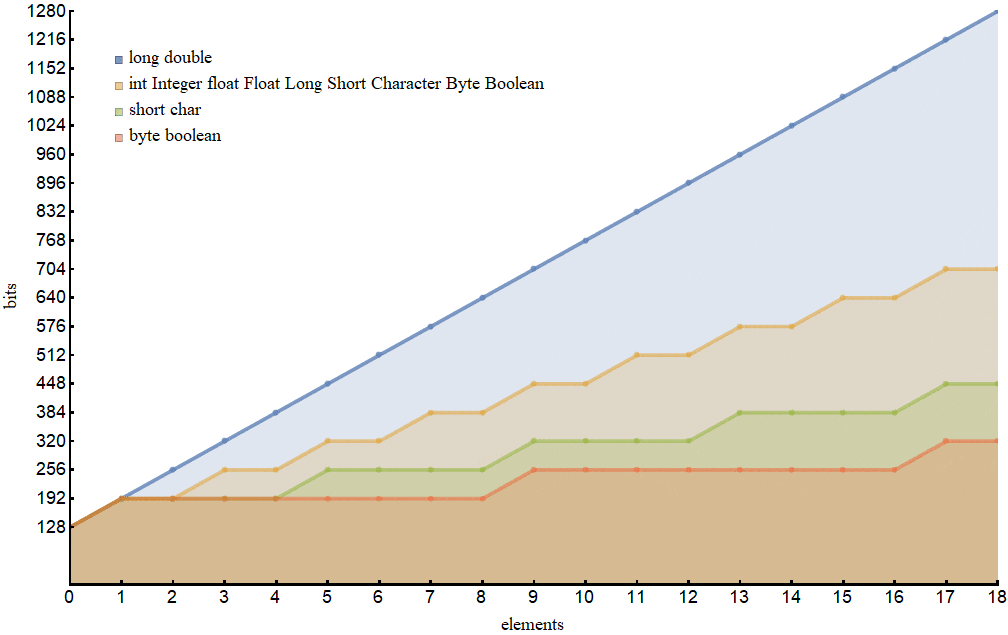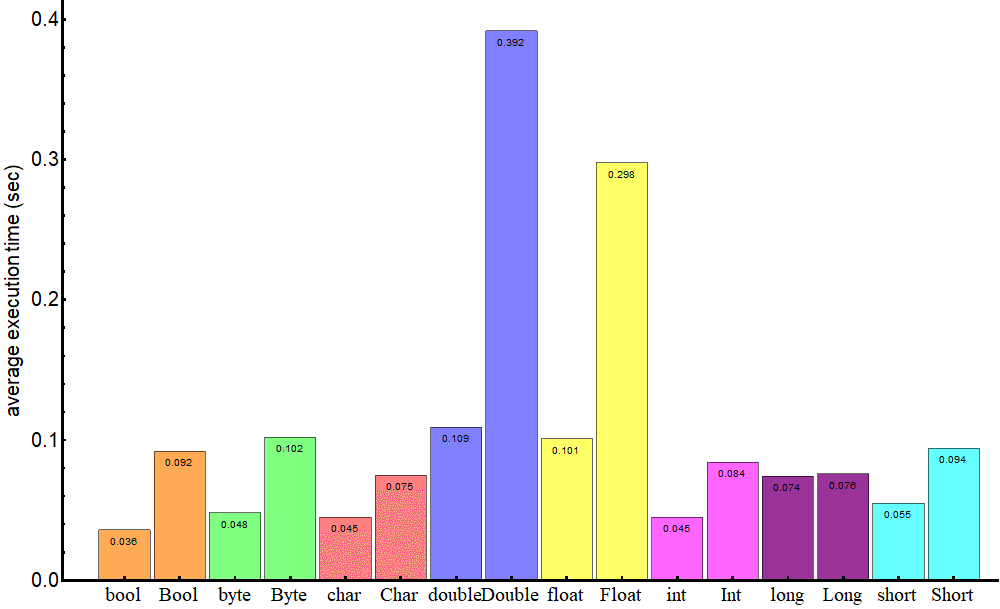### I just announced the new Learn Spring course, focused on the fundamentals of Spring 5 and Spring Boot 2:

>> CHECK OUT THE COURSE

## 1. Overview

In this tutorial, we show the pros and cons of using Java primitive types and their wrapped counterparts.

## 2. Java Type System

Java has a two-fold type system consisting of primitives such as int, boolean and reference types such as Integer, Boolean. Every primitive type corresponds to a reference type.

Every object contains a single value of the corresponding primitive type. The wrapper classes are immutable (so that their state can't change once the object is constructed) and are final (so that we can't inherit from them).

Under the hood, Java performs a conversion between the primitive and reference types if an actual type is different from the declared one:

``````Integer j = 1;          // autoboxing
int i = new Integer(1); // unboxing
``````

The process of converting a primitive type to a reference one is called autoboxing, the opposite process is called unboxing.

## 3. Pros and Cons

The decision what object is to be used is based on what application performance we try to achieve, how much available memory we have, the amount of available memory and what default values we should handle.

If we face none of those, we may ignore these considerations though it's worth knowing them.

### 3.1. Single Item Memory Footprint

Just for the reference, the primitive type variables have the following impact on the memory:

• boolean – 1 bit
• byte – 8 bits
• short, char – 16 bits
• int, float – 32 bits
• long, double – 64 bits

In practice, these values can vary depending on the Virtual Machine implementation. In Oracle's VM, the boolean type, for example, is mapped to int values 0 and 1, so it takes 32 bits, as described here: Primitive Types and Values.

Variables of these types live in the stack and hence are accessed fast. For the details, we recommend our tutorial on the Java memory model.

The reference types are objects, they live on the heap and are relatively slow to access. They have a certain overhead concerning their primitive counterparts.

The concrete values of the overhead are in general JVM-specific. Here, we present results for a 64-bit virtual machine with these parameters:

``````java 10.0.1 2018-04-17
Java(TM) SE Runtime Environment 18.3 (build 10.0.1+10)
Java HotSpot(TM) 64-Bit Server VM 18.3 (build 10.0.1+10, mixed mode)``````

To get an object's internal structure, we may use the Java Object Layout tool (see our another tutorial on how to get the size of an object).

It turns out that a single instance of a reference type on this JVM occupies 128 bits except for Long and Double which occupy 192 bits:

• Boolean – 128 bits
• Byte – 128 bits
• Short, Character – 128 bits
• Integer, Float – 128 bits
• Long, Double – 192 bits

We can see that a single variable of Boolean type occupies as much space as 128 primitive ones, while one Integer variable occupies as much space as four int ones.

### 3.2. Memory Footprint for Arrays

The situation becomes more interesting if we compare how much memory occupy arrays of the types under consideration.

When we create arrays with the various number of elements for every type, we obtain a plot:that demonstrates that the types are grouped into four families with respect to how the memory m(s) depends on the number of elements s of the array:

• long, double: m(s) = 128 + 64 s
• short, char: m(s) = 128 + 64 [s/4]
• byte, boolean: m(s) = 128 + 64 [s/8]
• the rest: m(s) = 128 + 64 [s/2]

where the square brackets denote the standard ceiling function.

Surprisingly, arrays of the primitive types long and double consume more memory than their wrapper classes Long and Double.

We can see either that single-element arrays of primitive types are almost always more expensive (except for long and double) than the corresponding reference type.

### 3.3. Performance

The performance of a Java code is quite a subtle issue, it depends very much on the hardware on which the code runs, on the compiler that might perform certain optimizations, on the state of the virtual machine, on the activity of other processes in the operating system.

As we have already mentioned, the primitive types live in the stack while the reference types live in the heap. This is a dominant factor that determines how fast the objects get be accessed.

To demonstrate how much the operations for primitive types are faster than those for wrapper classes, let's create a five million element array in which all elements are equal except for the last one; then we perform a lookup for that element:

``````while (!pivot.equals(elements[index])) {
index++;
}``````

and compare the performance of this operation for the case when the array contains variables of the primitive types and for the case when it contains objects of the reference types.

We use the well-known JMH benchmarking tool (see our tutorial on how to use it), and the results of the lookup operation can be summarized in this chart:Even for such a simple operation, we can see that it's required more time to perform the operation for wrapper classes.

In case of more complicated operations like summation, multiplication or division, the difference in speed might skyrocket.

### 3.4. Default Values

Default values of the primitive types are 0 (in the corresponding representation, i.e. 0, 0.0d etc) for numeric types, false for the boolean type, \u0000 for the char type. For the wrapper classes, the default value is null.

It means that the primitive types may acquire values only from their domains, while the reference types might acquire a value (null) that in some sense doesn't belong to their domains.

Though it isn't considered a good practice to leave variables uninitialized, sometimes we might assign a value after its creation.

In such a situation, when a primitive type variable has a value that is equal to its type default one, we should find out whether the variable has been really initialized.

There's no such a problem with a wrapper class variables since the null value is quite an evident indication that the variable hasn't been initialized.

## 4. Usage

As we've seen, the primitive types are much faster and require much less memory. Therefore, we might want to prefer using them.

On the other hand, current Java language specification doesn't allow usage of primitive types in the parametrized types (generics),  in the Java collections or the Reflection API.

When our application needs collections with a big number of elements, we should consider using arrays with as more “economical” type as possible, as it's illustrated on the plot above.

## 5. Conclusion

It this tutorial, we illustrated that the objects in Java are slower and have a bigger memory impact than their primitive analogs.

As always, code snippets can be found in our repository on GitHub.

### I just announced the new Learn Spring course, focused on the fundamentals of Spring 5 and Spring Boot 2:

>> CHECK OUT THE COURSE
Inline FeedbacksHurelhuyag
2 years ago

I think boolean is 8bit

2 years ago